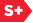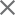### Proceedings Paper

Class of smooth nonseparable N-dimensional scaling functions
Author(s): Mohsen Maesumi
Format Member Price Non-Member Price
PDF \$17.00 \$21.00

Paper Abstract

Wavelets of compact support are an important tool in many areas of signal analysis. A starting point for the construction of such wavelets is the scaling function, the solution of the dilation equation. We study the dilation equation (phi) (X) equals (Sigma) KCK(phi )(2X-K) where K (epsilon) {0...m}N, (phi) :RN yields R, CK (epsilon) R. This paper gives a set of sufficient conditions on CK under which the solution of the dilation equation has a specific degree of regularity. We will construct (phi) through infinite products of 2N associated matrices with entries in terms of CK. The conditions for regularity are based on certain sum rules that triangularize all of the associated matrices and on certain inequalities that control the eigenvalues of the matrices. The net effect of the sum rules is to specify the coefficients CK in terms of a binomial interpolation of their values at the corners of the N-cube, {0,m}N. The inequalities are based on sums of the coefficients at the corners of the various faces of the N-cube. The number of derivatives that (phi) possesses and the Holder exponent of the last derivative can be determined for the sums.

Paper Details

Date Published: 11 October 1994
PDF: 11 pages
Proc. SPIE 2303, Wavelet Applications in Signal and Image Processing II, (11 October 1994); doi: 10.1117/12.188810
Show Author Affiliations
Mohsen Maesumi, Lamar Univ. (United States)

Published in SPIE Proceedings Vol. 2303:
Wavelet Applications in Signal and Image Processing II
Andrew F. Laine; Michael A. Unser, Editor(s)